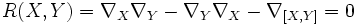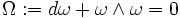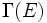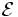# Flat connection

(diff) ← Older revision | Latest revision (diff) | Newer revision → (diff)

## Definition

### Symbol-free definition

A connection on a vector bundle over a differential manifold is said to be flat or integrable or curvature-free or locally flat if the curvature of the connection is zero everywhere.

### Definition with symbols

A connection$\nabla$ on a vector bundle$E$ over a differential manifold$M$ is said to be flat or integrable or curvature-free or locally flat if the curvature form vanishes identically, viz for any vector fields$X$ and$Y$:$R(X,Y) = \nabla_X\nabla_Y - \nabla_Y\nabla_X - \nabla_{[X,Y]} = 0$

### Definition in local coordinates

In local coordinates, we require that the curvature matrix should vanish identically; in other words:$\Omega := d\omega + \omega \wedge \omega = 0$

where$\omega$ is the matrix of connection forms.

### Alternative definitions

Further information: Flat connection equals module structure over differential operators

Recall that one alternative view of a connection is as giving the space of sections$\Gamma(E)$ the structure of a module over the connection algebra of$M$. Equivalently, it is a way of giving the sheaf of sections$\mathcal{E}$ the structure of a sheaf-theoretic module over the sheaf of connection algebras.

The connection is flat if and only if this descends to a module structure over the sheaf of differential operators. In other words, a flat connection is equivalent to a structure of$\mathcal{E}$ as a module over the sheaf of differential operators.